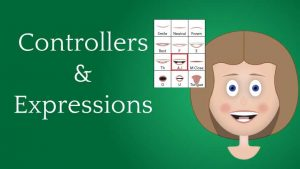Video updated: 30 November 20018

# Video 8 of the Controllers & Expressions Series: Rigging Angie’s eyes, part 3 of 5

In this video we’re going to connect the eyes to the Eye Control (X Y Controller handle) to give life to Angie’s eyes. Then we’ll add a couple of  slider controls so that we can modify the eye’s positions in interesting ways.

##Rigging Angie’s eyes,
part 3 of 5

If you missed part one, it’s here, along with the work files.

Video content:
• 0:00 Introduction
• 0:26  Cleaning up the timeline tabs
• 0:49 Setting up the interface
• 1:46 Connecting the L Eye Iris layer to the Eye Control
• 3:53 Using the clamp( ) expression to modify the Eye Control output
• 5:34 Connect the R Eye Iris layer, then adding the slider controls
• 8:02 Replace L Eye Iris Layer expression and modify
• 8:39 Showing slider effects & closing
• 9:16 End Credits

## Code from the video

The code below is provided as a reference, and was copied directly from the video After Effects file. Expression fields that contained only a pick whip connection are not included.

L Eye comp, L Iris position property

```IDX=comp("Angie Head v3").layer("Eye Control Handle").effect("Iris Distance X")("Slider");
IDY=comp("Angie Head v3").layer("Eye Control Handle").effect("Iris Distance Y")("Slider");
SP=[100,150]; //Starting Position
P=SP+clamp(EC,[-50,-60],[50,60]);
MP=P-[IDX,IDY];  //Modified Position by sliders
MP```

R Eye comp, R Pupil position property

```IDX=comp("Angie Head v3").layer("Eye Control Handle").effect("Iris Distance X")("Slider");
IDY=comp("Angie Head v3").layer("Eye Control Handle").effect("Iris Distance Y")("Slider");
SP=[100,150]; //Starting Position
P=SP+clamp(EC,[-50,-60],[50,60]);
MP=P+[IDX,IDY];  //Modified Position by sliders
MP```

Shortening the expressions

This expressions could be shorten and to do so is up to personal preference. I tend to be verbose so my process is clear. Below is an example of cutting out some steps of the L Eye comp expression. Also, instead of putting the starting position as an array [100,150] in line 4 (line 3 above), I could have substituted the term value which adopts the value of the property when the expression is not enabled .

```IDX=comp("Angie Head v3").layer("Eye Control Handle").effect("Iris Distance X")("Slider");
IDY=comp("Angie Head v3").layer("Eye Control Handle").effect("Iris Distance Y")("Slider");
P=[100,150]+clamp(EC,[-50,-60],[50,60])-[IDX,IDY];
P```

Or, here’s the extreme short version.

`[100,150]+clamp(comp("Angie Head v3").layer("Eye Control Handle").transform.position,[-50,-60],[50,60])+[comp("Angie Head v3").layer("Eye Control Handle").effect("Iris Distance X")("Slider"),comp("Angie Head v3").layer("Eye Control Handle").effect("Iris Distance Y")("Slider")];`

## Using the linear( ) expression instead of clamp( )

While the linear( ) expression could have been used instead of the clamp( ) expression, it would have taken a bit more lines of code. The linear expression can use target values that are array’s, but value source has to be a single number. This means I had to modify the EC variable’s X and Y output separately using the linear expression on lines 5 and 6, assigning the variables ECX and ECY respectively. I then added those X, Y modifications to the starting position in line 7, replacing the clamp( ) part of that expression.

L Eye comp, R Iris, position property

```IDX=comp("Angie Head v3").layer("Eye Control Handle").effect("Iris Distance X")("Slider");
IDY=comp("Angie Head v3").layer("Eye Control Handle").effect("Iris Distance Y")("Slider");
SP=[100,150]; //Starting Position
ECX=linear(EC,-100,100,-50,50); //Modify EC's X extreme values
ECY=linear(EC,-100,100,-60,60); //Modify EC's Y extreme values
P=SP+[ECX,ECY];
MP=P+[IDX,IDY];  //Modified Position by sliders
MP```

R Eye comp, R Pupil position property

```IDX=comp("Angie Head v3").layer("Eye Control Handle").effect("Iris Distance X")("Slider");
IDY=comp("Angie Head v3").layer("Eye Control Handle").effect("Iris Distance Y")("Slider");
SP=[100,150]; //Starting Position